# Classification Decision Rules

## Producer Field Guide

HGD_Product
Producer Field Guide
HGD_Portfolio_Suite
Producer

Once a set of reliable signatures has been created and evaluated, the next step is to perform a classification of the data. Each pixel is analyzed independently. The measurement vector for each pixel is compared to each signature, according to a decision rule, or algorithm. Pixels that pass the criteria that are established by the decision rule are then assigned to the class for that signature. Use ERDAS IMAGINE to classify the data both parametrically with statistical representation, and nonparametrically as objects in feature space. The "Classification Flow Diagram" below shows the flow of an image pixel through the classification decision making process in ERDAS IMAGINE (Kloer, 1994).

If a nonparametric rule is not set, then the pixel is classified using only the parametric rule. All of the parametric signatures are tested. If a nonparametric rule is set, the pixel is tested against all of the signatures with nonparametric definitions. This rule results in the following conditions:

• If the nonparametric test results in one unique class, the pixel is assigned to that class.
• If the nonparametric test results in zero classes (that is, the pixel lies outside all the nonparametric decision boundaries), then the unclassified rule is applied. With this rule, the pixel is either classified by the parametric rule or left unclassified.
• If the pixel falls into more than one class as a result of the nonparametric test, the overlap rule is applied. With this rule, the pixel is either classified by the parametric rule, processing order, or left unclassified.

Nonparametric Rules

ERDAS IMAGINE provides these decision rules for nonparametric signatures:

• Parallelepiped
• Feature Space

Unclassified Options

ERDAS IMAGINE provides these options if the pixel is not classified by the nonparametric rule:

• Parametric rule
• Unclassified

Overlap Options

ERDAS IMAGINE provides these options if the pixel falls into more than one feature space object:

• Parametric rule
• By order
• Unclassified

Parametric Rules

ERDAS IMAGINE provides these commonly-used decision rules for parametric signatures:

• Minimum Distance
• Mahalanobis Distance
• Maximum Likelihood (with Bayesian variation)
• Spectral Angle Mapper
• Spectral Correlation Mapper

Classification Flow Diagram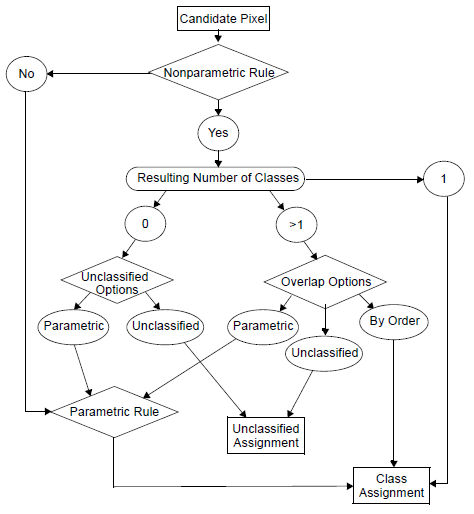Parallelepiped

In Parallelepiped decision rule, the data file values of the candidate pixel are compared to upper and lower limits. These limits can be either:

• minimum and maximum data file values of each band in the signature,
• mean of each band, plus and minus a number of standard deviations, or
• any limits that you specify, based on your knowledge of the data and signatures. This knowledge may come from the signature evaluation techniques discussed above.

These limits can be set using Parallelepiped Limits dialog in Signature Editor.

There are high and low limits for every signature in every band. When a pixel’s data file values are between the limits for every band in a signature, then the pixel is assigned to that signature’s class. The diagram below is a two-dimensional example of a parallelepiped classification.

Parallelepiped Classification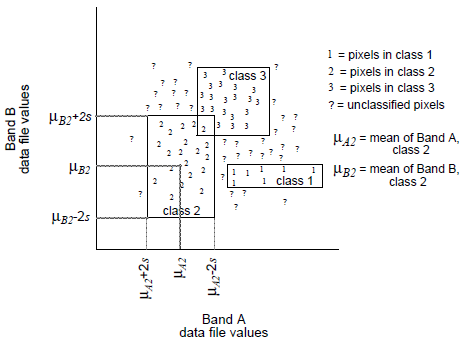The large rectangles in the figure above are called parallelepipeds. They are the regions within the limits for each signature.

Overlap Region

In cases where a pixel may fall into the overlap region of two or more parallelepipeds, you must define how the pixel can be classified.

• Classify the pixel by the order of the signatures. If one of the signatures is first and the other signature is fourth, the pixel is assigned to the first signature’s class. This order can be set in the Signature Editor.
• Classify the pixel by the defined parametric decision rule. The pixel is tested against the overlapping signatures only. If neither of these signatures is parametric, then the pixel is left as unclassified. If only one of the signatures is parametric, then the pixel is automatically assigned to that signature’s class.
• Leave the pixel as unclassified.

Regions Outside of the Boundaries

If the pixel does not fall into one of the parallelepipeds, then you must define how the pixel can be classified.

• Classify the pixel by the defined parametric decision rule. The pixel is tested against all of the parametric signatures. If none of the signatures is parametric, then the pixel is left as unclassified.
• Leave the pixel as unclassified.

Use Supervised Classification dialog in Signature Editor to perform a parallelepiped classification.

 Advantages Disadvantages Fast and simple, since the data file values are compared to limits that remain constant for each band in each signature. Since parallelepipeds have corners, pixels that are actually quite far, spectrally, from the mean of the signature may be classified. An example of this is shown in the figure below.. Often useful for a first-pass, broad classification, this decision rule quickly narrows down the number of possible classes to which each pixel can be assigned before the more time-consuming calculations are made, thus cutting processing time (for example, Minimum Distance, Mahalanobis Distance, or Maximum Likelihood). Not dependent on normal distributions.

Parallelepiped Corners Compared to the Signature Ellipse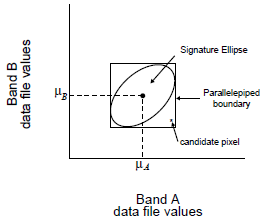Feature Space

Feature Space decision rule determines whether or not a candidate pixel lies within the nonparametric signature in the feature space image. When a pixel’s data file values are in the feature space signature, then the pixel is assigned to that signature’s class. The figure below is a two-dimensional example of a feature space classification. The polygons in this figure are AOIs used to define the feature space signatures.

Feature Space Classification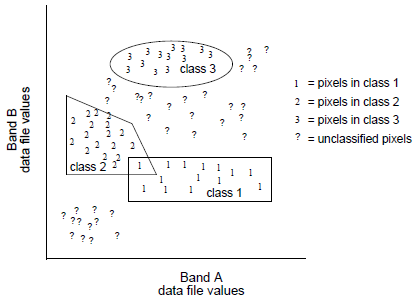Overlap Region

In cases where a pixel may fall into the overlap region of two or more AOIs, you must define how the pixel can be classified.

• Classify the pixel by the order of feature space signatures. If one of the signatures is first and the other signature is fourth, the pixel is assigned to the first signature’s class. This order can be set in the Signature Editor.
• Classify the pixel by the defined parametric decision rule. The pixel is tested against the overlapping signatures only. If neither of these feature space signatures is parametric, then the pixel is left as unclassified. If only one of the signatures is parametric, then the pixel is assigned automatically to that signature’s class.
• Leave the pixel as unclassified.

Regions Outside of the AOIs

If the pixel does not fall into one of the AOIs for the feature space signatures, then you must define how the pixel can be classified.

• Classify the pixel by the defined parametric decision rule. The pixel is tested against all of the parametric signatures. If none of the signatures is parametric, then the pixel is left as unclassified.
• Leave the pixel as unclassified.

Use Create Feature Space Images or Create Thematic Feature Space Images dialog in Signature Editor to perform a feature space classification.

 Advantages Disadvantages Often useful for a first-pass, broad classification. Feature Space decision rule allows overlap and unclassified pixels. Provides an accurate way to classify a class with a nonnormal distribution (for example, residential and urban). Feature space image may be difficult to interpret. Certain features may be more visually identifiable, which can help discriminate between classes that are spectrally similar and hard to differentiate with parametric information. Feature space method is fast.

Minimum Distance

Minimum Distance decision rule (also called spectral distance) calculates the spectral distance between the measurement vector for the candidate pixel and the mean vector for each signature.

Minimum Spectral Distance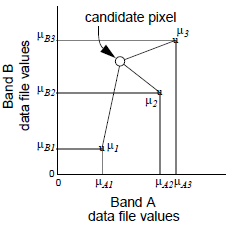In the figure above, spectral distance is illustrated by the lines from the candidate pixel to the means of the three signatures. The candidate pixel is assigned to the class with the closest mean.

The equation for classifying by spectral distance is based on the equation for Euclidean distance: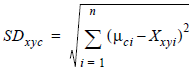Where:

n = number of bands (dimensions)

i = a particular band

c = a particular class

Xxyi = data file value of pixel x,y in band i

mci = mean of data file values in band i for the sample for class c

SDxyc = spectral distance from pixel x,y to the mean of class c

Source: Swain and Davis, 1978

When spectral distance is computed for all possible values of c (all possible classes), the class of the candidate pixel is assigned to the class for which SD is the lowest.

 Advantages Disadvantages Since every pixel is spectrally closer to either one sample mean or another, there are no unclassified pixels. Pixels that should be unclassified (that is, they are not spectrally close to the mean of any sample, within limits that are reasonable to you) become classified. However, this problem is alleviated by thresholding out the pixels that are farthest from the means of their classes. Fastest decision rule to compute, except for parallelepiped. Does not consider class variability. For example, a class like an urban land cover class is made up of pixels with a high variance, which may tend to be farther from the mean of the signature. Using this decision rule, outlying urban pixels may be improperly classified. Inversely, a class with less variance, like water, may tend to overclassify (that is, classify more pixels than are appropriate to the class), because the pixels that belong to the class are usually spectrally closer to their mean than those of other classes to their means.

Mahalanobis Distance

Mahalanobis Distance is similar to Minimum Distance, except that the covariance matrix is used in the equation. Variance and covariance are figured in so that clusters that are highly varied lead to similarly varied classes, and vice versa. For example, when classifying urban areas—typically a class whose pixels vary widely—correctly classified pixels may be farther from the mean than those of a class for water, which is usually not a highly varied class (Swain and Davis, 1978).

Mahalanobis Distance algorithm assumes that the histograms of the bands have normal distributions. If this is not the case, you may have better results using Parallelepiped or Minimum Distance decision rule, or by performing a first-pass parallelepiped classification.

The equation for the Mahalanobis Distance classifier is as follows: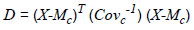Where:

D = Mahalanobis Distance

c = a particular class

X = measurement vector of the candidate pixel

Mc = mean vector of the signature of class c

Covc = covariance matrix of the pixels in the signature of class c

Covc-1 = inverse of Covc

T = transposition function

The pixel is assigned to the class, c, for which D is the lowest.

 Advantages Disadvantages Takes the variability of classes into account, unlike Minimum Distance or Parallelepiped. Tends to overclassify signatures with relatively large values in the covariance matrix. If there is a large dispersion of the pixels in a cluster or training sample, then the covariance matrix of that signature contains large values. May be more useful than Minimum Distance in cases where statistical criteria (as expressed in the covariance matrix) must be taken into account, but the weighting factors that are available with the Maximum Likelihood / Bayesian option are not needed. Slower to compute than Parallelepiped or Minimum Distance. Mahalanobis Distance is parametric, meaning that it relies heavily on a normal distribution of the data in each input band.

Maximum Likelihood/Bayesian

Maximum Likelihood decision rule is based on the probability that a pixel belongs to a particular class. The basic equation assumes that these probabilities are equal for all classes, and that the input bands have normal distributions.

Maximum Likelihood algorithm assumes that the histograms of the bands of data have normal distributions. If this is not the case, you may have better results with the Parallelepiped or Minimum Distance decision rule, or by performing a first-pass parallelepiped classification.

Bayesian Classifier

If you have a priori knowledge that the probabilities are not equal for all classes, you can specify weight factors for particular classes. This variation of the Maximum Likelihood decision rule is known as the Bayesian decision rule (Hord, 1982). Unless you have a priori knowledge of the probabilities, it is recommended that they not be specified. In this case, these weights default to 1.0 in the equation.

The equation for the Maximum Likelihood/Bayesian classifier is as follows: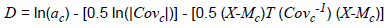Where:

D = weighted distance (likelihood)

c = a particular class

X = measurement vector of the candidate pixel

Mc =mean vector of the sample of class c

ac =percent probability that any candidate pixel is a member of class c (defaults to 1.0, or is entered from a priori knowledge)

Covc = covariance matrix of the pixels in the sample of class c

|Covc| = determinant of Covc (matrix algebra)

Covc-1 = inverse of Covc (matrix algebra)

ln = natural logarithm function

T = transposition function (matrix algebra)

The inverse and determinant of a matrix, along with the difference and transposition of vectors, would be explained in a textbook of matrix algebra.

The pixel is assigned to the class, c, for which D is the lowest.

 Advantages Disadvantages Most accurate of the classifiers in ERDAS IMAGINE system (if the input samples / clusters have a normal distribution), because it takes the most variables into consideration. An extensive equation that takes a long time to compute. The computation time increases as the number of input bands increases. Takes the variability of classes into account by using the covariance matrix, as does Mahalanobis Distance. Maximum Likelihood is parametric, meaning that it relies heavily on a normal distribution of the data in each input band. Tends to overclassify signatures with relatively large values in the covariance matrix. If there is a large dispersion of the pixels in a cluster or training sample, then the covariance matrix of that signature contains large values.

Spectral Angle Mapper

Spectral Angle Mapper algorithm derives the angle formed between a reference spectrum and the image spectrum of each pixel. The spectral angle is a calculation that permits rapid comparison of the spectral similarity of two spectra. Each spectrum is treated as a vector in N-dimensional space where N is equal to the number of bands in the image. In the context of supervised classification, the reference spectrum is the mean vector for each signature.

In the optional output Distance File (grayscale) image from Supervised Classification or Threshold operations, the results are mapped as the sum of the angles of the two spectral curves.

In this presentation, values vary between zero (0) and pi/2 (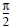) where 0 represents a best match. Darker pixels indicate a smaller angular distance and, therefore, a better match to the reference spectrum.

Spectral Correlation Mapper

This algorithm is a modified Spectral Angler Mapper approach where each spectrum is normalized by the spectrum mean.

In the optional output Distance File (grayscale) image from Supervised Classification or Threshold operations, the results are mapped as the sum of the angles of the two spectral curves.

In this presentation, values vary between zero (0) and pi/2 (), where 0 represents a best match. Darker pixels indicate a smaller angular distance and, therefore, a better match to the reference spectrum.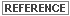Spectral Angle Mapper and Spectral Correlation Mapper algorithms are described in ERDAS IMAGINE Help Spectral Analysis User Guide.